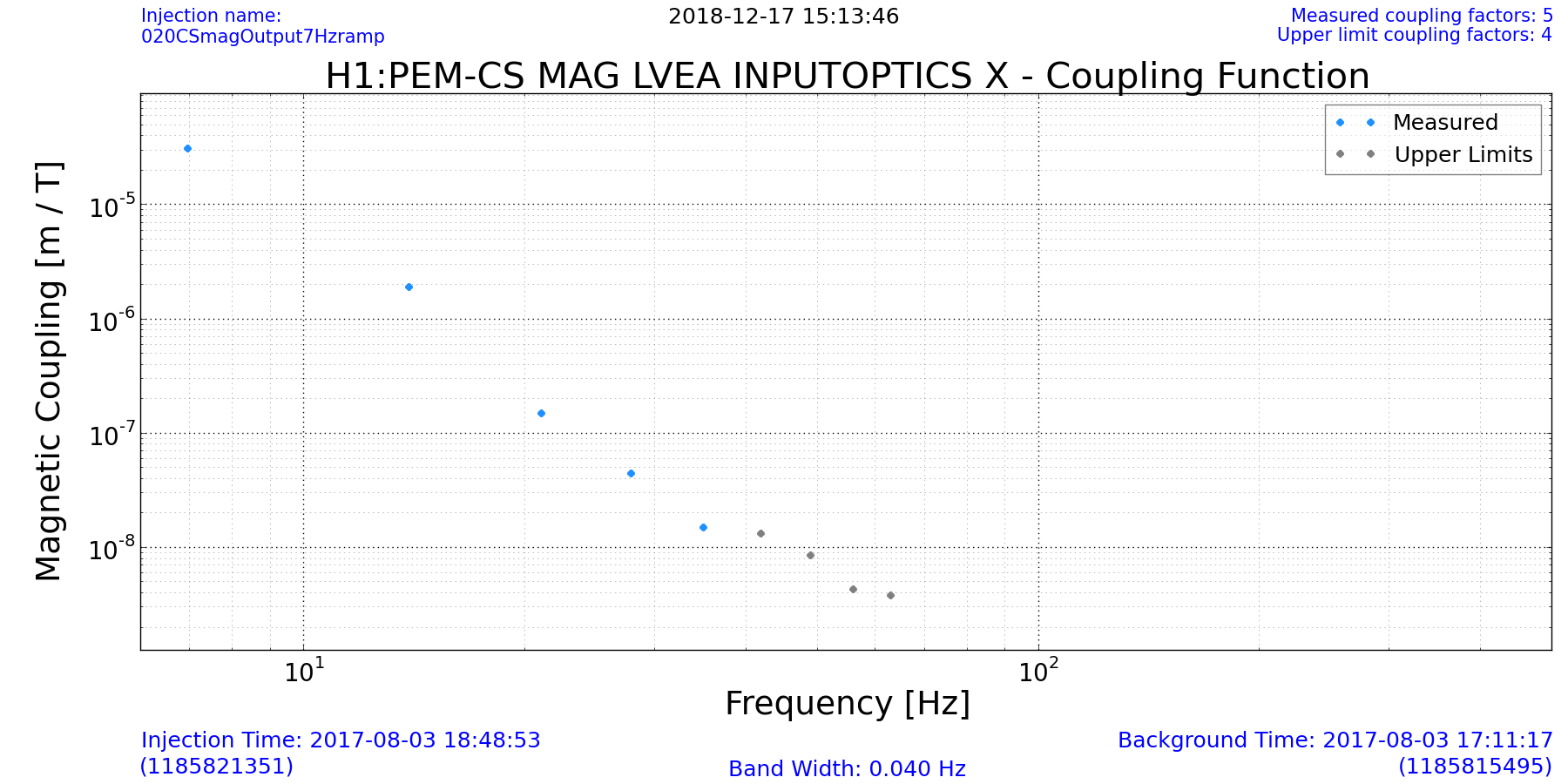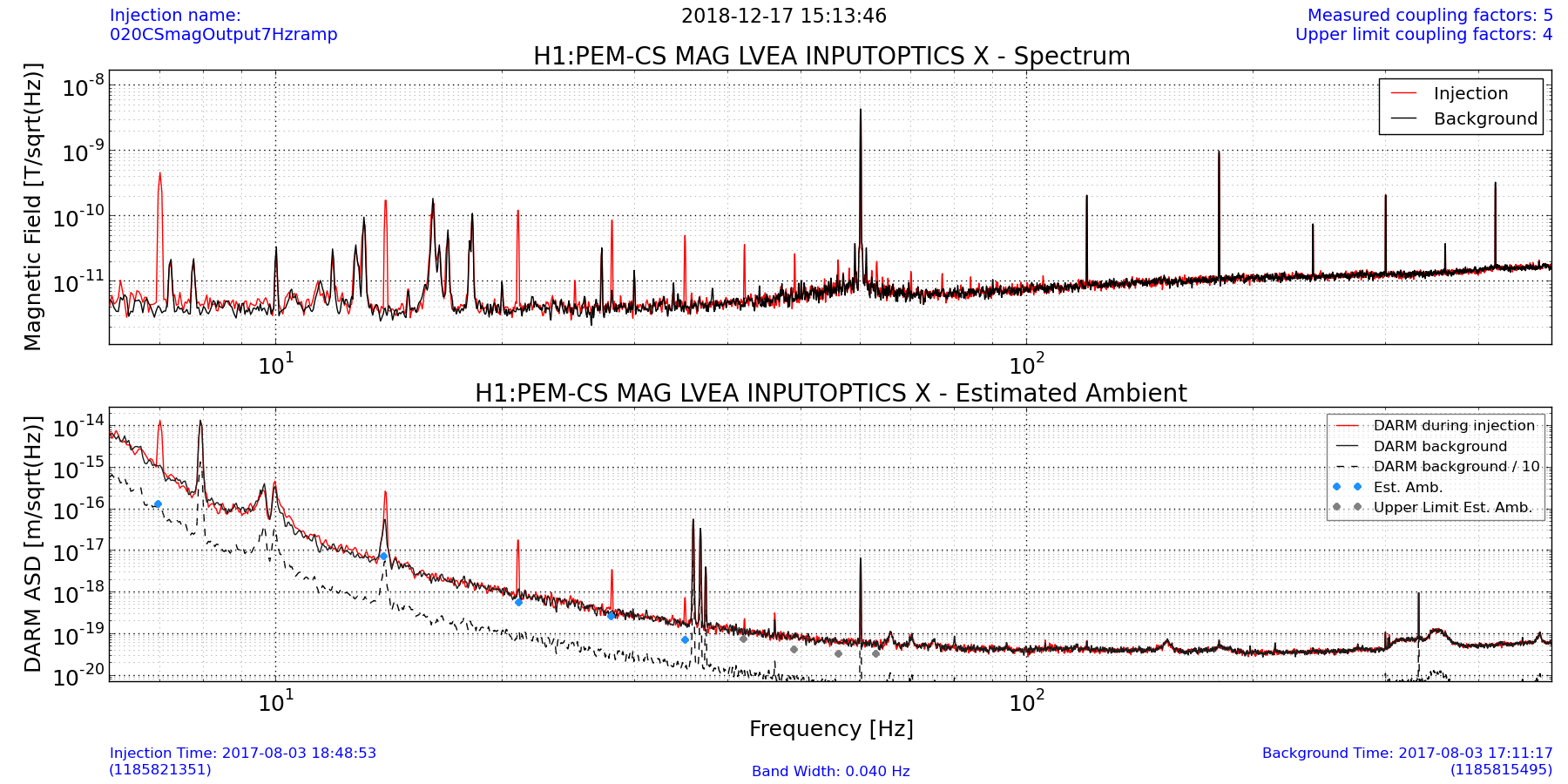# Coupling functions¶

## Thresholds¶

Measuring a coupling function requires a significant increase in amplitude spectral density in both DARM and the sensor. Each value of a coupling function is flagged based on a sensor threshold and DARM threshold. When the sensor ASD exceeds the sensor threshold and the DARM ASD exceeds the DARM threshold, the value is a measurement. When only the sensor ASD exceeds its thresholds, the value is an upper limit.

## Calculation¶

A measured value is computed as

$$CF(f) = \sqrt{\frac{Y_{inj}^2(f) - Y_{bkg}^2(f)}{X_{inj}^2(f) - X_{bkg}^2(f)}}$$

where $$X_{inj}(f)$$ and $$X_{bkg}(f)$$ are the values of the sensor ASD during injection and background, and $$Y_{inj}(f)$$ and $$Y_{bkg}(f)$$ are the values of the DARM ASD during injection and background

An upper limit is computed as

$$CF(f) = \frac{Y_{bkg}(f)}{\sqrt{X_{inj}^2(f) - X_{bkg}^2(f)}}$$

Below is an example plot of a coupling function for a magnetometer at LHO.## Estimated ambient¶

Multiplying coupling function by a sensor background spectrum gives an estimated ambient in DARM for that sensor.

Below is an example plot of a spectrum and estimated ambient plot for a magnetometer at LHO.